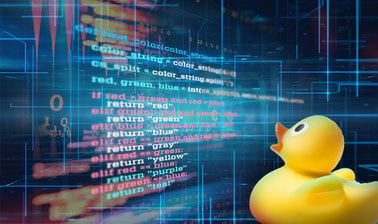# Computing in Python I: Fundamentals and Procedural Programming

Learn the fundamentals of computing in Python, including variables, operators, and writing and debugging your own programs.After a course session ends, it will be archived.
Estimated 5 weeks
9–10 hours per week
Self-paced

This course starts from the beginning, covering the basics of how a computer interprets lines of code; how to write programs, evaluate their output, and revise the code itself; how to work with variables and their changing values; and how to use mathematical, boolean, and relational operators.

By the end of this course, you'll be able to write small programs in Python that use variables, mathematical operators, and logical operators. For example, you could write programs that carry out complex mathematical operations, like calculating the interest rate necessary to reach a savings goal, recommending apparel options based on weather patterns, or calculating a grade based on multiple percentages.

Structurally, the course is comprised of several parts. Instruction is delivered via a series of short (2-3 minute) videos. In between those videos, you'll complete both multiple choice questions and coding problems to demonstrate your knowledge of the material that was just covered.

### At a glance

• Institution: GTx
• Subject: Computer Science
• Level: Introductory
• Prerequisites:

This course presupposes no prior knowledge beyond basic arithmetic; knowledge of high school-level algebra, however, is desirable.

# What you'll learn

Skip What you'll learn
• How a computer processes programming code
• The write-run-debug cycle of writing code, running it, and revising it based on its output.
• Procedural programming, or how to write sequential lines of code.
• Variables, their types, and their role in complex programs.
• Mathematical operators for arithmetic operations, exponents, and more.
• Relational operators for evaluating relative values or set membership.
• Boolean operators for resolving complex logical statements.

# Syllabus

Skip Syllabus

Chapter 1: Computing. The fundamentals of how computers work, what program code is, and how to get setup for the rest of the course.

Chapter 2: Programming. The basic principles of computer programming: writing and running code, evaluating results, and compiling vs. executing.

Chapter 3: Debugging. The common results of running program code, and how to use those results to inform revision of your code.

Chapter 4: Procedural Programming. The fundamental approach to program code: writing sequences of lines of code that run in order to accomplish an objective.

Chapter 5: Variables. Creating and modifying variables, tracing how their values may change as a program runs, and understanding the role of data types.

Chapter 6: Logical Operators. Working with relational (greater than, less than, equal to) and logical (and, or, not) operators to make decisions in code.

Chapter 7: Mathematical Operators. Adding addition, subtraction, multiplication, division, modulus, and exponents to your code, and seeing how they work with unexpected data types.